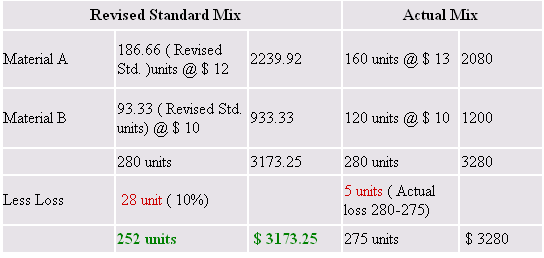222

## Latest \$type=blogging\$count=5\$author=hide\$comment=hide\$label=hide\$date=hide\$show=home

Material yield variance is the part of material variances. It shows the variance due to difference in actual abnormal loss and standard abnormal loss.  It is excess of material quantity variance over material mix variance. If we deduct material mix variance from material quantity variance, we can calculate material yield variance. With following examples we are explaining material yield variance.

1st Example : When standard mix and actual mix of material are same :

From the following data, calculate materials yield variance :

Standard Mix

Material A 200 units @ \$ 12

Material B 100 units @ \$ 10

Actual Mix

160 units @ \$ 13

140 units @ \$ 10

Standard loss allowed is 10% of input. Actual output is 275 units.

In this case standard and actual mix do not differ, So there is no need of calculating revised standard mix.

 Standard Mix Actual Mix Material A 200 units @ \$ 12 2400 160 units @ \$ 13 2080 Material B 100 units @ \$ 10 1000 140 units @ \$ 10 1400 300 units 3400 300 units 3480 Less Loss 30 unit ( 10%) 25 units ( Actual loss 300-275) 270 units \$ 3400 275 units \$ 3480

Standard Cost per unit = \$ 3400 / 270= \$ 12.593

Material Yield Variance = Standard Rate ( Actual Yield - Standard Yield )

= \$ 12.593 ( 275 - 270) = \$ 62.965 Favourable

Material Yield Variance's 1st  Example Simplified :

I know many of readers of Accounting Education could not understand this example. Friends ! \$ 62.965 is favourable material yield variance. You know that yield means output. We (Company) did best. Our actual output is more than standard output. You also know, when does it happen? It happens only when we have succeeded to reduce our abnormal losses. In above example, we fixed standard or estimated abnormal loss 10% of total standard quantity of A and B material. But we did our work more efficiently. After this, our actual abnormal loss occurs only  8.33% of actual quantity. Due to this reason, our material yield variance is favourable for our business's growth.

Verification

Material Yield Variance = Material Quantity Variance - Material Mix Variance

It means for verification of material yield variance, we have to calculate material quantity variance and material mix variance. With this verification, you will also learn the difference between material mix variance and material yield variance.

Material Quantity Variance

= Standard Price ( Standard Quantity of one type of material/ (total standard material - Abnormal Loss ) X actual output - Actual Quantity)

MQV for material A = \$ 12 ( 200/270 X 275-160) = \$ 524.44 Favourable

MQV for material B = \$ 10 ( 100/270X275 -140) = \$ 381.48 Unfavourable

--------------------------------------------------------------------------------
Total MQV = \$ 142.96 favourable
===========================================

Material Mix Variance

MMV  =standard cost of standard mix - standard cost actual mix

MMV = \$ 3400 - (\$ 12 X 160 + \$ 10 X 140)

MMV = (\$ 80) favourable

MYV = MQV - MMV = 62.96 Favourable

2nd Example : When standard mix and actual mix of material are not same :

To understand material yield variance is little difficult when standard mix and actual mix of material are not same. But if you will seriously study and remember the formula, you can use it easily.

Calculate Material Yield Variance from following information

Standard Mix

Material A 200 units @ \$ 12

Material B 100 units @ \$ 10

Actual Mix

160 units @ \$ 13

120 units @ \$ 10

Standard loss allowed is 10% of input. Actual output is 275 units.

In this case standard and actual mix are 300 units and 280 units which are different, So there is the need of calculating revised standard mix.

Revised Standard Mix of A material

= Standard Quantity of A / Total Standard Quantity of A and B X Total Actual Quantity of A and B material

= 200/ 300 X 280 = 186.66 units

Same way, we will calculate revised standard mix of B material

= 100 / 3000 X 280 = 93.33 units

Standard Rate per unit = \$ 3173.25/ 252 =\$ 12.59

Material Yield Variance = Std. Rate ( Actual Yield - Standard Yield)

= \$ 12.59 ( 275 -252) = \$ 289.57 Favourable because actual production is more than standard production.

Related : Material Mix Variance Examples: 1
1.very useful....keep it up

In comment, you can give your feedback, reviews, ideas for improving content or ask question relating to written content.

Name

ltr
item
Accounting Education: Material Yield Variance Examples
Material Yield Variance Examples
http://2.bp.blogspot.com/-0nEXdi2GheE/TfhE4Q4DDbI/AAAAAAAAGoI/QUQm5_njMHA/s1600/myv.PNG
http://2.bp.blogspot.com/-0nEXdi2GheE/TfhE4Q4DDbI/AAAAAAAAGoI/QUQm5_njMHA/s72-c/myv.PNG
Accounting Education
https://www.svtuition.org/2011/06/material-yield-variance-examples.html
https://www.svtuition.org/
https://www.svtuition.org/
https://www.svtuition.org/2011/06/material-yield-variance-examples.html
true
2410664366776677676
UTF-8